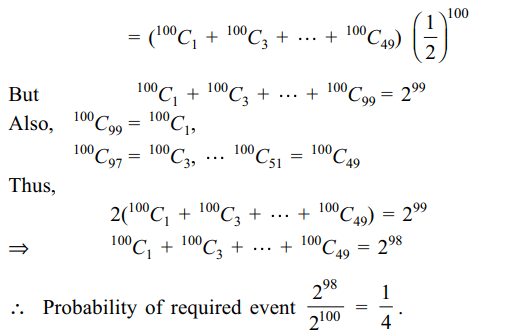## Probability Questions and Answers Part-3

1. A computer producing factory has only two plants $T_{1}$ and $T_{2}$  . Plant $T_{1}$ produces 20% and plant $T_{2}$ produces 80% of the total computers produced. 7% of computers produced in the factory turn out of be defective. It is known that
P(computer turns out to be defective given that it is produced in plant $T_{1}$) = 10 P (computer turns out to be defective given that it is produced in plant $T_{2}$)
where P(E) denotes the probability of an event E. A computer produced in the factory is randomly selected and it does not turn out to be defective. Then the probability that it is produced in plant $T_{2}$ is
a) $\frac{36}{73}$
b) $\frac{47}{79}$
c) $\frac{78}{93}$
d) $\frac{75}{83}$

Explanation: Let T1, T2, D denote the following events.2. Seven distinct white balls and three distinct black balls are randomly placed in a row. The probability that no two black balls are placed adjacently equals
a) 1/2
b) 7/15
c) 2/15
d) 1/3

Explanation: The number of ways of placing 3 black balls at3. If from each of the three boxes containing 3 white and 1 black, 2 white and 2 black, 1 white and 3 black balls, one ball is drawn at random, then the probability that two white and one black ball will be drawn is
a) $\frac{13}{32}$
b) $\frac{1}{4}$
c) $\frac{1}{32}$
d) $\frac{3}{16}$

Explanation: P (2 white and 1 black)4. There are four machines and it is known that exactly two of them are faulty. They are tested, one by one, in a random order till both the faulty machines are identified , then the probability that only two tests are needed is
a) $\frac{1}{3}$
b) $\frac{1}{6}$
c) $\frac{1}{2}$
d) $\frac{1}{4}$

Explanation: Let A(B) denote the event that each of the first5. A bag contains some white and some black balls, all combinations of balls being equally likely. The total number of balls in the bag is 10. If three balls are drawn at random without replacement and all of them are found to be black, the probability that the bag contains 1 white and 9 black balls is
a) $\frac{14}{55}$
b) $\frac{12}{55}$
c) $\frac{2}{11}$
d) $\frac{8}{55}$

Explanation: Let Ei denote the event that the bag contains i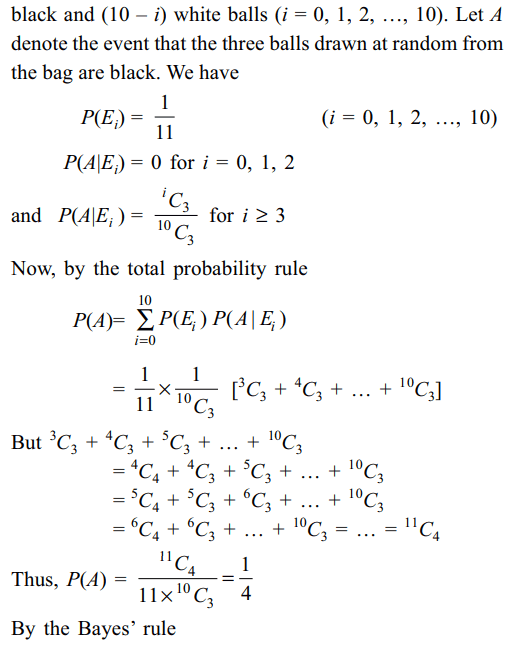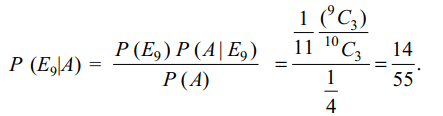6. A group of 2n boys and 2n girls is randomly divided into two equal groups. The probability that each group contains the same number of boys and girls is
a) 1/2
b) 1/n
c) $\frac{^{2n}C_{n}}{^{4n}C_{n}}$
d) $\frac{\left(^{2n}C_{n}\right)^{2}}{^{4n}C_{n}}$

Explanation: Total number of ways of choosing a group is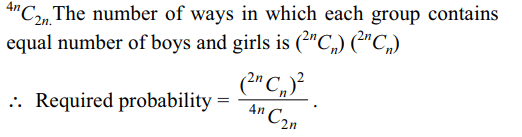7. An unbiased die with faces marked 1, 2, 3, 4, 5 and 6 is rolled four times out of the four faces obtained, the probability that the minimum value is exactly than 2 and the maximum value is exactly 5 is
a) 4/27
b) 1/81
c) 80/81
d) 65/81

Explanation: Total number of outcomes of 4 dice is 64. We8. Four fair dice $D_{1},D_{2},D_{3}$   and $D_{4}$ , each having six faces numbered 1, 2, 3, 4, 5 and 6 are rolled simultaneously. The probability that $D_{4}$ shows a number appearing on one of $D_{1},D_{2},$  and $D_{3}$ is
a) $\frac{91}{216}$
b) $\frac{108}{216}$
c) $\frac{125}{216}$
d) $\frac{127}{216}$

Explanation: Probability of the required event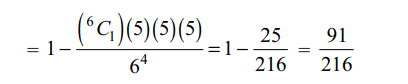9. A pair of unbiased dice is rolled together till a sum of either 5 or 7 is obtained. The probability that 5 comes before 7 is
a) 2/5
b) 3/5
c) 4/5
d) 1/5